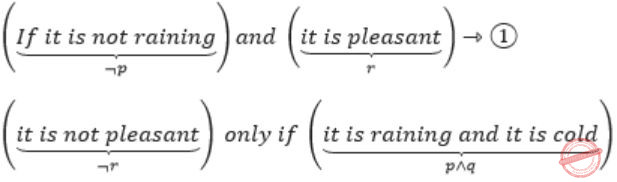## Prepositional-Logic

 Question 1
Consider the first-order logic sentence F: ∀x(∃yR(x,y)). Assuming non-empty logical domains, which of the sentences below are implied by F?
I. ∃y(∃xR(x,y))
II. ∃y(∀xR(x,y))
III. ∀y(∃xR(x,y))
IV. ¬∃x(∀y¬R(x,y))
 A IV only B I and IV only C II only D II and III only
Engineering-Mathematics       Prepositional-Logic       GATE 2017 [Set-1]       Video-Explanation
Question 1 Explanation:
Lets convert the given first order logic sentence into some english sentence.
F: ∀x(∃yR(x,y)) (given)
: For all girls there exist a boyfriend
(x for girls and y for boys)
I: ∃y(∃xR(x,y))
: There exist some boys who have girlfriends.
(Subset of statement F, so True)
II: ∃y(∀xR(x,y))
: There exists some boys for which all the girls are girlfriend. (False)
III: ∀y(∃xR(x,y))
: For all boys exists a girlfriend. (False)
IV: ~∃x(∀y~R(x,y))
= ∀x(~∀y~R(x,y))
= ∀x(∃yR(x,y)) (∵ ~∀y=∃y, ~∃x=∀x)
(True)
 Question 2

Let p, q and r be prepositions and the expression (p → q) → r be a contradiction. Then, the expression (r → p) → q is

 A a tautology B a contradiction C always TRUE when p is FALSE D always TRUE when q is TRUE
Engineering-Mathematics       Prepositional-Logic       GATE 2017 [Set-1]       Video-Explanation
Question 2 Explanation:
Given that (p→q)→r is a contradiction.
So r = F and (p→q) = T.
We have to evaluate the expression
(r→p)→q
Since r = F, (r→p) = T (As F→p, is always true)
The final expression is T→q and this is true when q is true, hence option D.
 Question 3

Let p, q, r denote the statements “It is raining”, “It is cold”, and “It is pleasant”, respectively. Then the statement “It is not raining and it is pleasant, and it is not pleasant only if it is raining and it is cold” is represented by

 A (¬p ∧ r) ∧ (¬r → (p ∧ q)) B (¬p ∧ r) ∧ ((p ∧ q) → ¬r) C (¬p ∧ r) ∨ ((p ∧ q) → ¬r) D (¬p ∧ r) ∨ (r → (p ∧ q))
Engineering-Mathematics       Prepositional-Logic       GATE 2017 [Set-2]       Video-Explanation
Question 3 Explanation:
p: It is raining
q: It is cold
r: It is pleasant
“If it is not raining and it is pleasant, and it is not pleasant only if it is raining and it is cold.”
We can divide the statement into two parts with “Conjunction”.i.e., ¬r→(p∧q) ⇾(2)
From (1) & (2), the given statement can be represented asQuestion 4
Let p,q,r,s represent the following propositions.
p: x ∈ {8,9,10,11,12}
q: x is a composite number
r: x is a perfect square<
s: x is a prime number
The integer x≥2 which satisﬁes ¬((p ⇒ q) ∧ (¬r ∨ ¬s))  is _________.
 A 11 B 12 C 13 D 14
Engineering-Mathematics       Prepositional-Logic       GATE 2016 [Set-1]       Video-Explanation
Question 4 Explanation:
Given,
~((p→q) ∧ (~r ∨ ~S))
⇒ first simplify the given statement by converging them to ∧, ∨
⇒ [~(p→q) ∨ (~(~r ∨ ~s)]
Demorgan’s law:
⇒ [~(~p ∨ q) ∨ (r ∧ s)]
∵ p→q ≡ ~p ∨ q
⇒ [(p ∧ ~q) ∨ (r ∧ s)]
p ∧ ~q is {8,9,10,11,12} ∧ {not a composite number} i.e. {11}
r ∧ s is {perfect square} ∧ {prime} i.e. no answer
So, the one and only answer is 11.
 Question 5

Consider the following statements:

S1: If a candidate is known to be corrupt, then he will not be elected.
S2: If a candidate is kind, he will be elected.

Which one of the following statements follows from S1 and S2 as per sound inference rules of logic?

 A If a person is known to corrupt, he is kind B If a person is not known to be corrupt, he is not kind C If a person is kind, he is not known to be corrupt D If a person is not kind, he is not known to be corrupt
Engineering-Mathematics       Prepositional-Logic       GATE 2015 [Set-2]
Question 5 Explanation:
Let p: candidate known to be corrupt
q: candidate will be elected
r: candidate is kind
then S1 = p→~q
= q→~p (conrapositive rule)
and S2: r→q ⇒ r→~p (transitive rule)
i.e., If a person is kind, he is not known to be corrupt. ∴ Option is C
 Question 6

In a room there are only two types of people, namely Type 1 and Type 2. Type 1 people always tell the truth and Type 2 people always lie. You give a fair coin to a person in that room, without knowing which type he is from and tell him to toss it and hide the result from you till you ask for it. Upon asking, the person replies the following:

`“The result of the toss is head if and only if I am telling the truth.”`

Which of the following options is correct?

 A The result is head B The result is tail C If the person is of Type 2, then the result is tail D If the person is of Type 1, then the result is tail
Engineering-Mathematics       Prepositional-Logic       GATE 2015 [Set-3]
Question 6 Explanation:
We do not know the type of person from whom those words are coming from and so we can have two cases.
Case 1:
The person who speaks truth. This definitely implies that result of toss is Head.
Case 2:
The person who lies. In this the reality will be the negation of the statement.
The negation of (x⇔y) is exactly one of x or y holds. "The result of the toss is head if and only if I am telling the truth". So here two possibilities are there,
→ It is head and lie spoken.
→ It is not head and truth spoken.
Clearly, the second one cannot speaks the truth. So finally it is head.
Hence, option (A).
There are 6 questions to complete.

Register Now